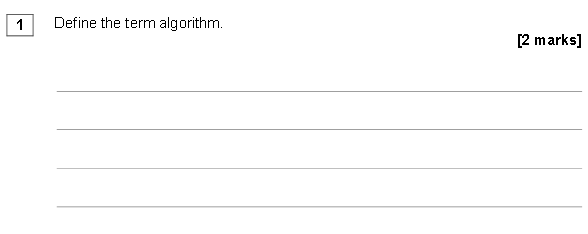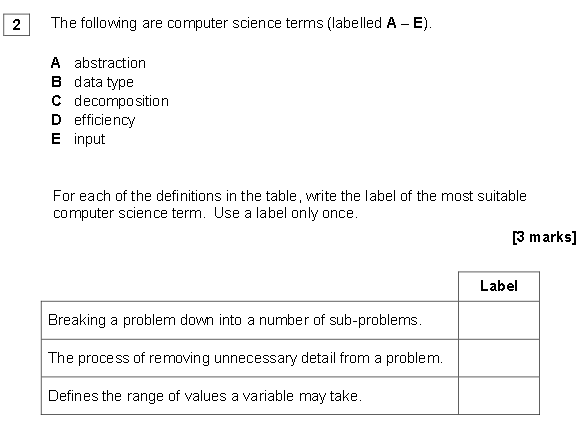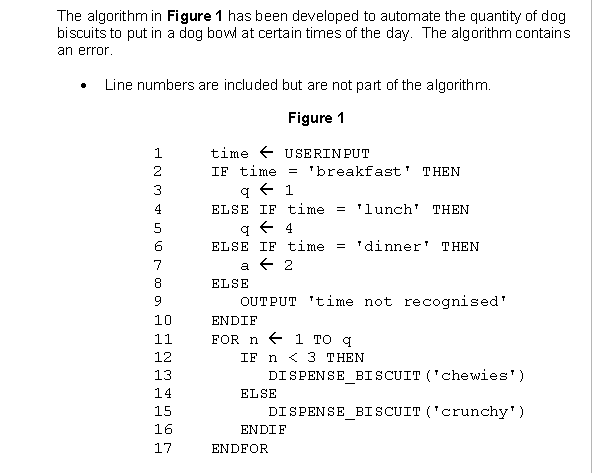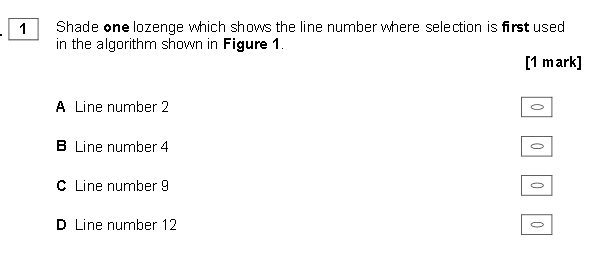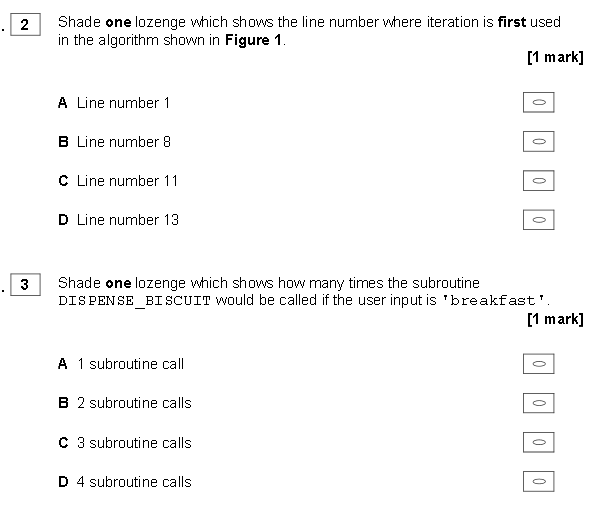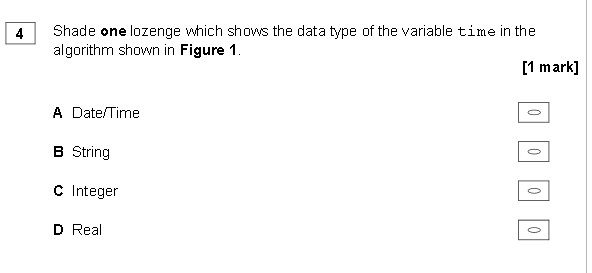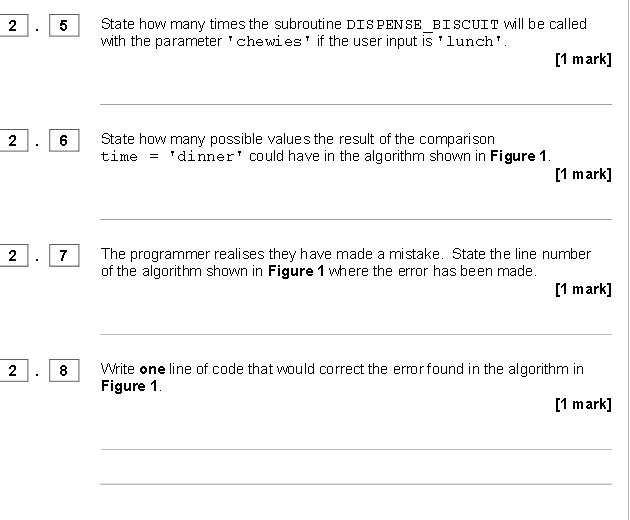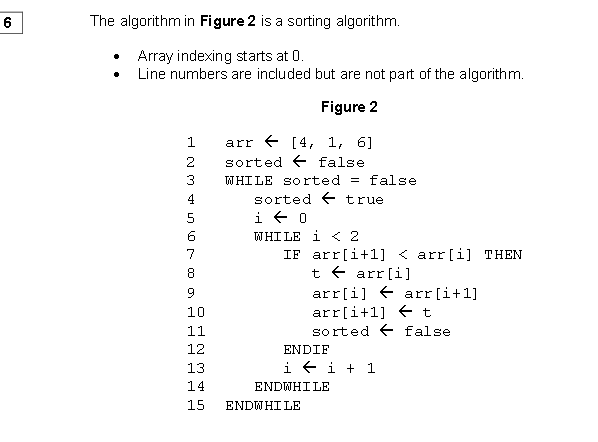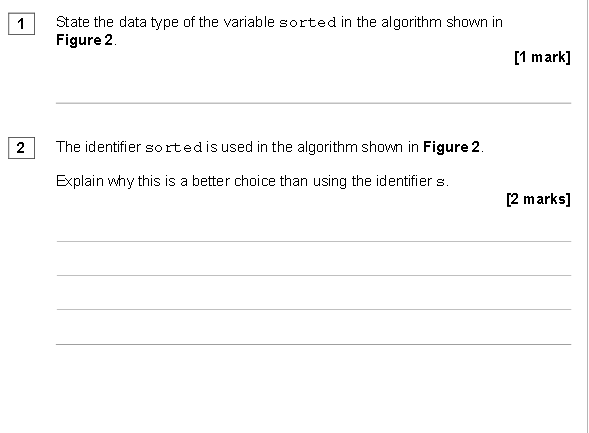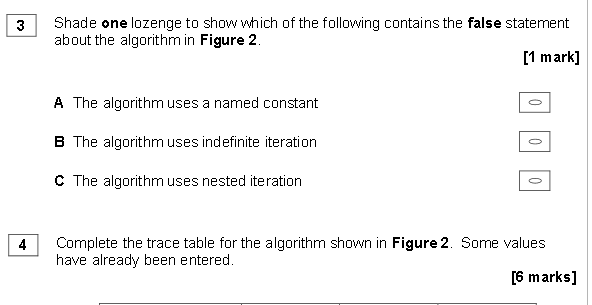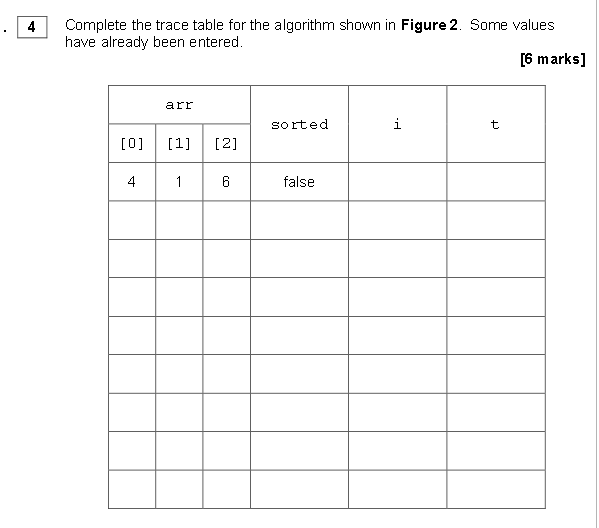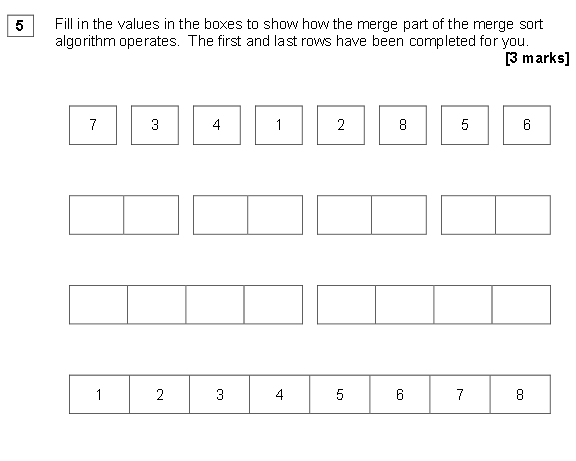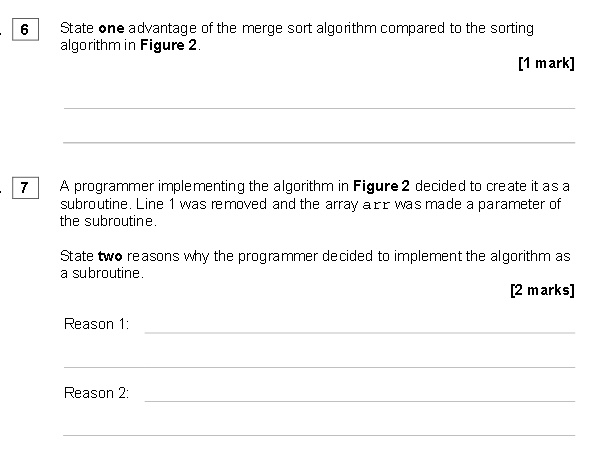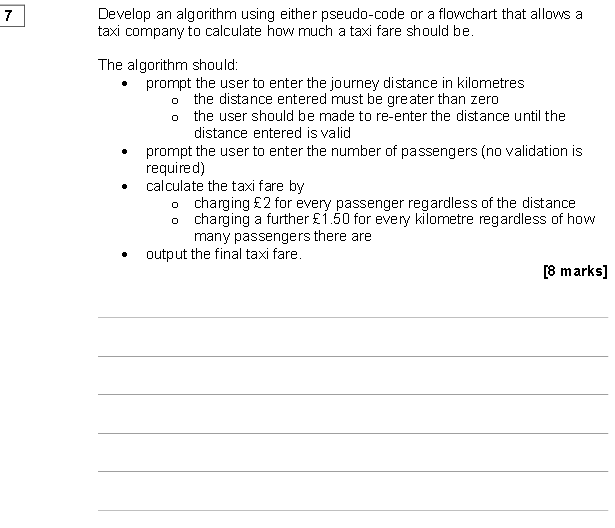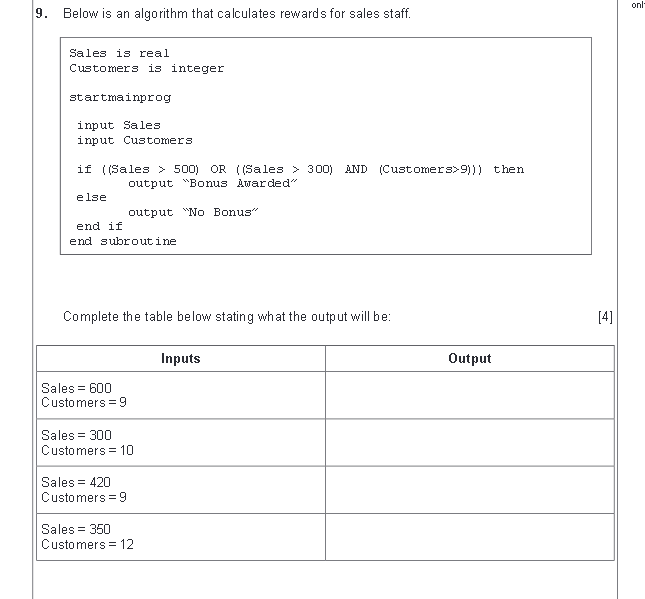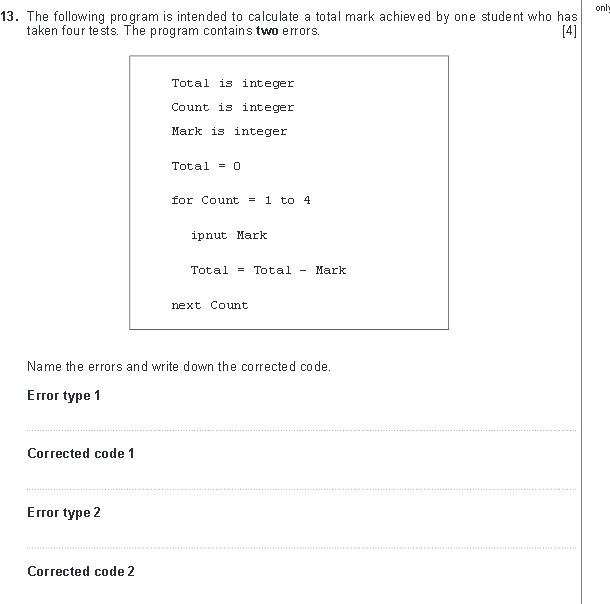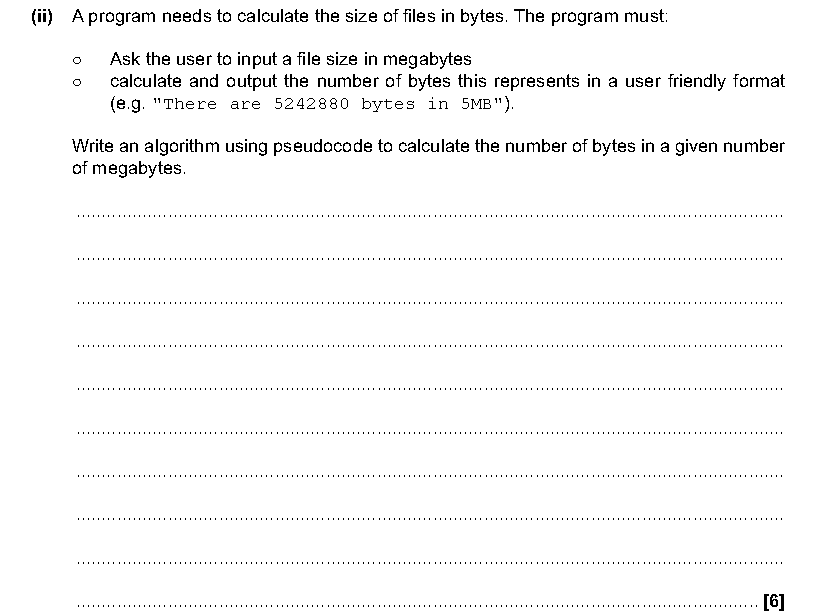# Theory: Problem Solving

This section looks at the problem solving section of the course. You need to create a powerpoint called 'Problem Solving' and all tasks and information need to be saved into this powerpoint. This will form the basis of your revision notes for this topic.

Algorithms

Algorithms provide a precise set of step by step instructions to solve a problem. All computer problems are algorithms. Algorithms improve efficiency by removing the need for human input. There are 3 constructs used in algorithms sequence, selection & iteration. Sequence relates to instructions being given in the correct order (eg enter a number). Selection relates to a decision being made and a course of action selected (eg IF statements). Iteration involves previous steps being repeated until a desired outcome is reached (eg for or while loops).

Task 1: Copy the code below into your revision notes and annotate what bits of the code relate to selection, sequence or iteration.

import time
import random
loaded=int(input("Enter a bullet into a chamber between 1-6:"))
spins=int(input("Enter the number of spins:"))
for i in range(spins):
print("Spin :",i+1)
time.sleep(2)
shot=(random.randint(1,6))
print("Random Chamber :",shot)
break
else:
pass
print("Game Over")

What is meant by the term selection?

What is meant by the term iteration?

What is meant by the term sequence?

What is an algorithm?

Write an algorithm in written text to guide someone to make a cup of tea.

Give 2 ways algorithms can be displayed.

Why might algorithms be reused?

Algorithms: Pseudo-code

Pseudo code is one way in which algorithms can be displayed. It uses structured english with commands and keywords similar to computer language. It is used to develop the logic of the algorithm without having to bother about computer syntax. Human's can read pseudo code even if it contains errors. A programmer can convert pseudo code into a programming language. The pseudo code below asks a user to enter their favourite subject.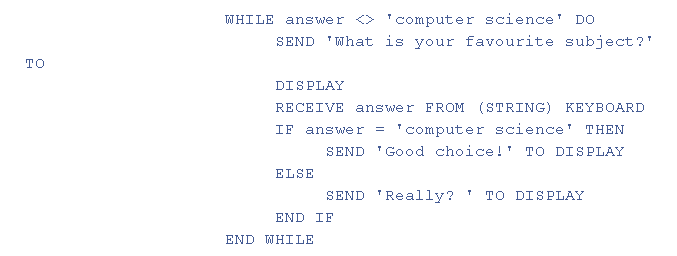• https://tools.withcode.uk/ks4pseudo/media/edexcel_pseudocode.pdf
• Write out the pseudo code for a program that asks a user for 12 numbers then returns the total.
• Explain what is meant by the term pseudo code.
• What are the advantages of using pseudo code?
• Write out the pseudo-code to convert an 8 digit binary number to a denary number.

Algorithms: Flow Charts

Algorithms can also be displayed as flow charts. The symbols used in flowcharts are shown below.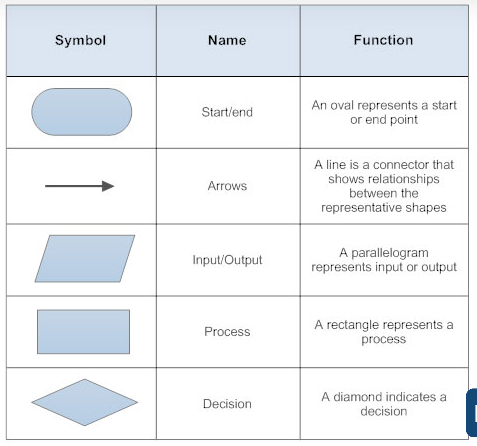Create a flowchart to work out the cost of sending parcels. If a parcel weighs 2kg or under it costs £2. If the parcel weighs over 2kg it costs £2 + £3 for every extra kg.

Create a flowchart to calculate the sum of 10 numbers if the total is over 100 the output needs to say pass, otherwise fail.

Create a flowchart for a dice game where 3 dice are thrown. if all dices are equal the score is the value of the dices shown doubled. if 2 dices match the score is the two matching values minus the third dice. If no dice match the score is zero.

Task: Use the internet to research model answers to the past paper questions below.....